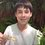# Sweet mental math trick

I was in my math class talking about something (probably a difference of two squares) and my math teacher showed us a cool trick to multiplying certain numbers. One example would be 35,45. First take the number In between these (40). Then get the amount needed for each number to equal 40 (5). Then multiply 40x40=1600 and get 5x5=25. Then 1600-25=1575. Pretty cool? Yes it is. Please tell me if you already knew that. I would love to catch up on these simple tricks.Note by Robert Fritz
7 years, 4 months ago

This discussion board is a place to discuss our Daily Challenges and the math and science related to those challenges. Explanations are more than just a solution — they should explain the steps and thinking strategies that you used to obtain the solution. Comments should further the discussion of math and science.

When posting on Brilliant:

• Use the emojis to react to an explanation, whether you're congratulating a job well done , or just really confused .
• Ask specific questions about the challenge or the steps in somebody's explanation. Well-posed questions can add a lot to the discussion, but posting "I don't understand!" doesn't help anyone.
• Try to contribute something new to the discussion, whether it is an extension, generalization or other idea related to the challenge.

MarkdownAppears as
*italics* or _italics_ italics
**bold** or __bold__ bold
- bulleted- list
• bulleted
• list
1. numbered2. list
1. numbered
2. list
Note: you must add a full line of space before and after lists for them to show up correctly
paragraph 1paragraph 2

paragraph 1

paragraph 2

[example link](https://brilliant.org)example link
> This is a quote
This is a quote
    # I indented these lines
# 4 spaces, and now they show
# up as a code block.

print "hello world"
# I indented these lines
# 4 spaces, and now they show
# up as a code block.

print "hello world"
MathAppears as
Remember to wrap math in $$ ... $$ or $ ... $ to ensure proper formatting.
2 \times 3 $2 \times 3$
2^{34} $2^{34}$
a_{i-1} $a_{i-1}$
\frac{2}{3} $\frac{2}{3}$
\sqrt{2} $\sqrt{2}$
\sum_{i=1}^3 $\sum_{i=1}^3$
\sin \theta $\sin \theta$
\boxed{123} $\boxed{123}$

Sort by:

Let the inbetween number be $\hat{r} = \frac{x+y}{2}$ so that

\begin{aligned} x &= \hat{r} + \frac{x-y}{2} \\ y &= \hat{r} - \frac{x-y}{2} \end{aligned}

where the difference between $x$ and the inbetween number (and also from $y$ to the inbetween number) is $\frac{x-y}{2}$

Then $\displaystyle xy = \left(\hat{r} + \frac{x-y}{2}\right)\left(\hat{r} - \frac{x-y}{2}\right) = \hat{r}^2 - \left(\frac{x-y}{2}\right)^2$, just like you say!

Staff - 7 years, 4 months ago

This is based on the identity $(a+b)(a-b) = a^2 - b^2$. I learnt this identity and started applying it to use some 2 years ago.

- 7 years, 4 months ago

Yeah pretty cool right, i learned it in 5 grade, but then I couldnot use algebra to justify it lack of Algebra

- 7 years, 4 months ago

It seems I'm far behind sweet mental math tricks.

- 7 years, 3 months ago

$(\frac{x+y}{2})^{2}-(\frac{x-y}{2})^{2} \Longrightarrow (\frac{x+y}{2}+\frac{x-y}{2})(\frac{x+y}{2}-\frac{x-y}{2}) \Longrightarrow (\frac{2x}{2})(\frac{2y}{2}) \Longrightarrow \boxed{xy}$ which is the desired result! Pretty awesome!

- 7 years, 4 months ago

The following is a trick to square a number ending with 5. For example take 35 then ignore 5 take rest of the number 3 in this case, now 3(3+1)=12 and 55=25 so the answer is 1225. Try it out with other cases

- 7 years, 3 months ago

Thank you! I am forever in your gratitude.

- 7 years, 3 months ago

We could have also multiplied in 10 s (3040)=1200 nd den (55)= 25 finally add 1200 +25 =1225

- 6 years, 9 months ago